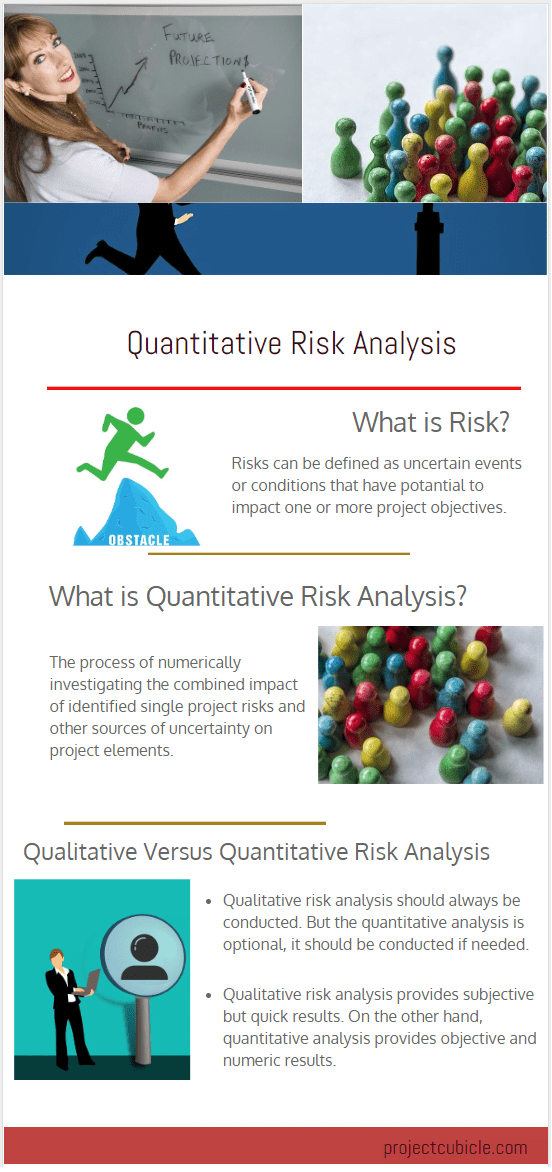During the life cycle of a project, team members encounter different types of risks. Those risks often have negative impacts on the project objectives. However, some of them have positive impacts. Risk analysis is an important concept in project management because as a project manager if you don’t know how to identify and evaluate factors that could affect the success of your project, you can not deal with them adequately. Therefore, project managers should have enough knowledge of conducting different types of risk analysis. Most of the time, qualitative risk analysis provides you enough understanding of the characteristics of a given risk. However, in some cases, you need to conduct a perform quantitative risk analysis process to evaluate the risk. In this article, we will discuss both quantitative and qualitative risk analysis definitions, tools and examples to help you to understand their key differences. Note that quantitative and qualitative risk analysis is a significant concept for PMP or CAPM Certification Exams.

### Definition of Risk

A risk can be defined in various aspects. In project management, risk is any unexpected event that has the potential to affect the project goals – positive or negative. Risks can influence resources, deliverables, processes and objectives of a project. Risks can be classified as positive or negative risks according to their impacts. Risk management process includes identification, evaluation, prioritization, and response.

## What is Quantitative Risk Analysis?

Quantitative risk analysis focuses on numerically evaluating the influence of project risks on project elements. It is performed to understand the probability and impact of risks on project objectives. For instance, in a construction project, quantitative risk analysis helps us to calculate the impact of a project delay such as obtaining a permit late causes twenty day delay with a cost of \$80,000.

Quantitative risk analysis is an objective tool, that quantifies project risks which are usually prioritized during qualitative risk analysis. It is difficult to perform this risk analysis for all project risks because of time constraints. Therefore it is often performed for the risks that have the highest probability and impact. The main purpose of conducting risk analysis is to determine the most appropriate strategies to deal with both positive and negative risks.

## What is the Purpose of Quantitative Risk Analysis

As discussed above, quantitative risk analysis is a numeric and objective risk analysis tool. It provides detailed information regarding the probability and impact of a given risk. From this aspect, it supports decision making. Considering critical business decisions, this tool provides more data and information than qualitative analysis.

It helps project managers and business owners to make better duration and cost estimates. This is an important point because, if the estimates are not correct, they will lead wrong decisions.

## When to Use Quantitative Risk Analysis

The process often flows as the following;

• Identifying project risks
• Scaling project risks by using qualitative risk analysis
• Performing quantitative risk analysis for the critical risks

So, when to use this risk analysis

• If your project requires Contingency Reserve to manage schedule and budget risks
• If your project is large and sophisticated and you need better decisions
• If you need more accurate and objective risk analysis for a given risk

## Difference Between Qualitative and Quantitative Risk Analysis

Below table summarizes the difference between these two risk analysis

 Qualitative Risk Analysis Quantitative Risk Analysis Focuses on all the risks identified in the identify risk process. Focuses on all the risks that have a possibility and high impact on the project elements. Does not use numerical methods. Uses mathematical calculations Scales risks by using numbers (0-5) or percentages. Calculates the effect of risk as a monetary value (cost) or number (duration). It is applied to all almost all projects It is often applied to large and complex projects

## Quantitative Risk Analysis Tools

There are many tools and techniques available to perform quantitative risk analysis. Below are a few of them;

• Decision Tree Analysis: A visual technique that supports decision making.
• Expected Monetary Value: A statistical technique that helps to compare and evaluate risks. It can be used along with decision tree analysis.
• Three-Point Estimate: Three-point estimate is applicable to both duration and cost estimates. It relies on three different estimates that are Optimistic, Pessimistic and Most Likely estimates.
• Sensitivity Analysis: Sensitivity analysis is also referred to as what-if or simulation analysis. It is a financial model that identifies how target variables are affected based on changes in other variables.
• Failure Mode and Effects Analysis (FMEA): is the process of analyzing as many components as possible to determine potential failure modes in a system and their causes and effects.
• Monte Carlo Analysis: Monte Carlo Analysis is performed to model the probability of various outcomes in a process that can’t handily be estimated because of the intervention of random variables. It is used along with three-point estimates.

## Quantitative Risk Analysis Example

In this risk analysis example, we will use the Expected Monetary Value technique to calculate the project risk exposure and the amount of Contingency Reserve.

 Risk Probability Cost Impact Expected Monetary Value (Probability x Impact) Risk 1 (Threat) 15% \$30.000,00 \$4.500,00 Risk 2 (Opportunity) 20% -\$20.000,00 -\$4.000,00 Risk 3 (Threat) 10% \$60.000,00 \$6.000,00 Total Expected Monetary Value \$6.500,00

### Conclusion

If you are preparing for your PMI PMP Certification Exam, you need to understand the basics of quantitative risk analysis definitions, tools, and examples. Because PMP aspirants often get confused about how quantitative and qualitative risk analysis differs. In this article, we explained their differences by focusing on quantitative risk analysis definition, tools and examples. If you want to add or share anything regarding the concept, please use the comments section below.

Cost Benefit Analysis Example